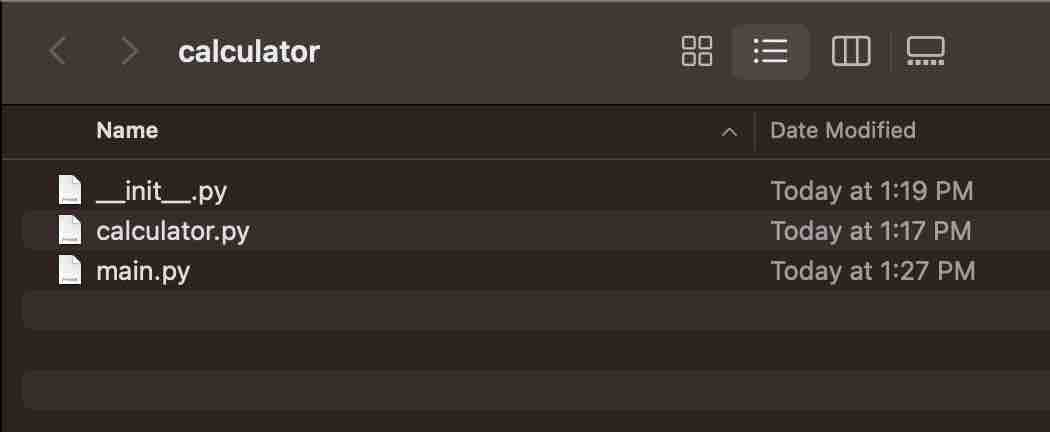# Import Other Python Files Examples

Say you have created a Python file calculator.py and you want to access this file from another Python file then follow the below steps.

## Step 1: Put the file inside a folder

Create a folder say calculator and add the calculator.py file in it.

``````def add(a, b):
return a + b

def subtract(a, b):
return a - b
``````

## Step 2: Create __init__.py

Next, create an empty __init__.py file within the same folder.

## Step 3: Create another py file.

Now let's see various ways to access the calculator.py file from main.py

Example 1: Import the entire module
``````import calculator.calculator

print(result)

result = calculator.calculator.subtract(8, 4)
print(result)
``````

Example 2: Import the entire module as alias
``````import calculator.calculator as calc

print(result)

result = calc.subtract(8, 4)
print(result)
``````
Example 3: Import specific functions from the module
``````from calculator.calculator import add, subtract

print(result)

result = subtract(8, 4)
print(result)
``````
Example 4: Import all functions from the module
``````from calculator.calculator import *

print(result)

result = subtract(8, 4)
print(result)
``````

Files Structure
``````calculator/
__init__.py
calculator.py
main.py
``````-

### Facing issues? Have Questions? Post them here! I am happy to answer!Author Info:

Rakesh is a seasoned developer with over 10 years of experience in web and app development, and a deep knowledge of operating systems. Author of insightful How-To articles for Code2care.